#Algebra 2 Worksheets

## Exponential & Logarithmic Functions Worksheets

Here is a graphic preview for all of the Exponential and Logarithmic Functions Worksheets. You can select different variables to customize these Exponential and Logarithmic Functions Worksheets for your needs. These Exponential and Logarithmic Functions Worksheets are randomly created and will never repeat so you have an endless supply of quality Exponential and Logarithmic Functions Worksheets to use in the classroom or at home.

Our Exponential and Logarithmic Functions Worksheets are free to download, easy to use, and very flexible.

These Exponential and Logarithmic Functions Worksheets are a good resource for students in the 8th Grade through the 12th Grade.

Click here for a Detailed Description of all the Exponential and Logarithmic Functions Worksheets.

## Quick Link for All Exponential and Logarithmic Functions Worksheets

Click the image to be taken to that Exponential and Logarithmic Functions Worksheets.

##### Exponents PropertiesHandout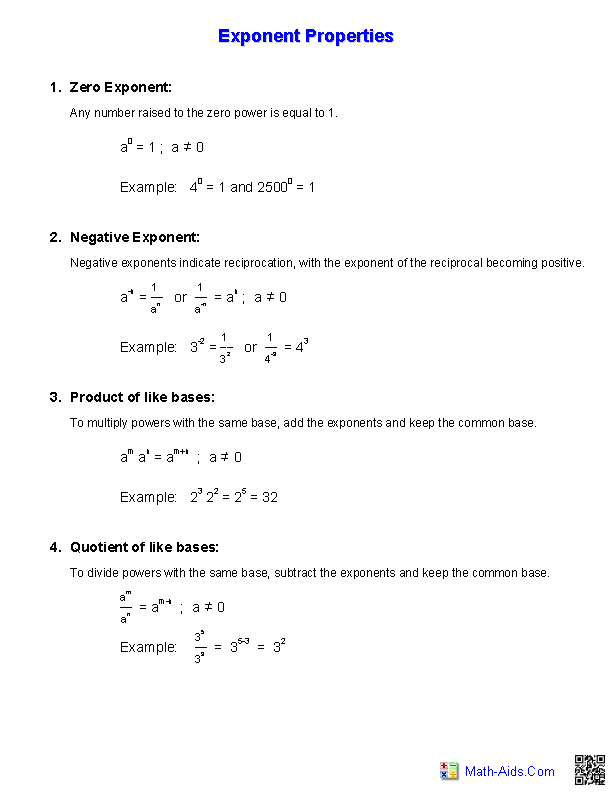##### The Meaning of LogarithmsWorksheets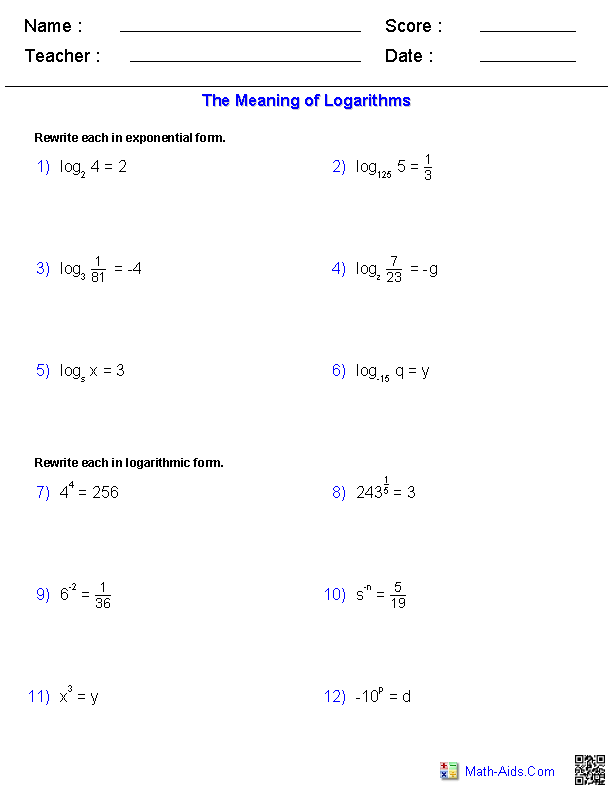##### Properties of LogarithmsWorksheets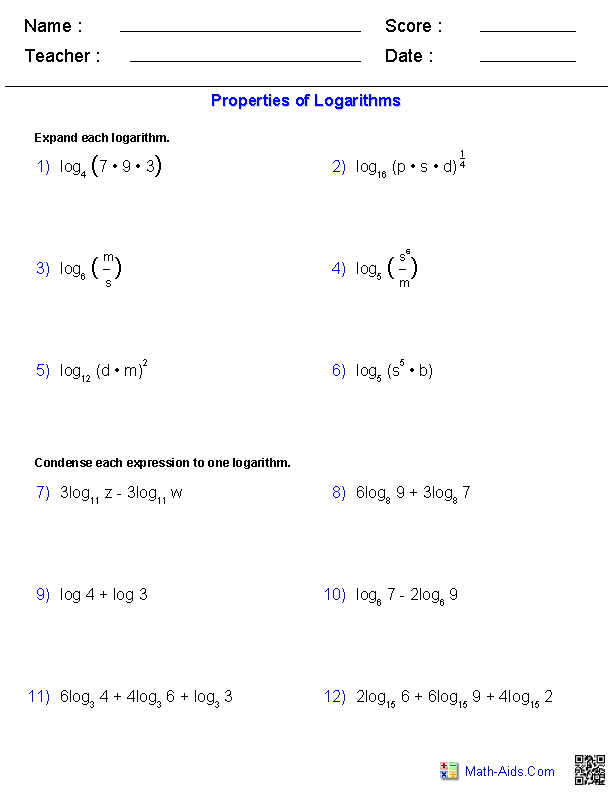##### The Change of Base FormulaWorksheets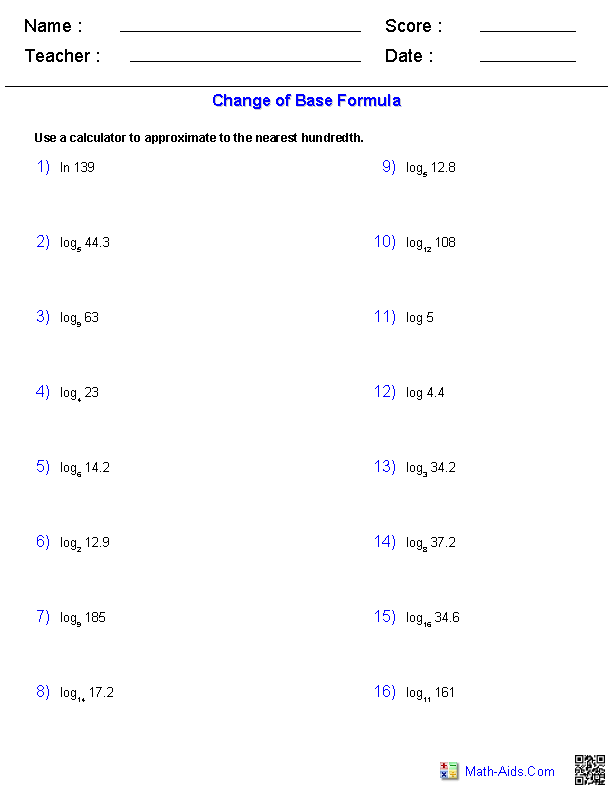##### Writing Logs inTerms of Others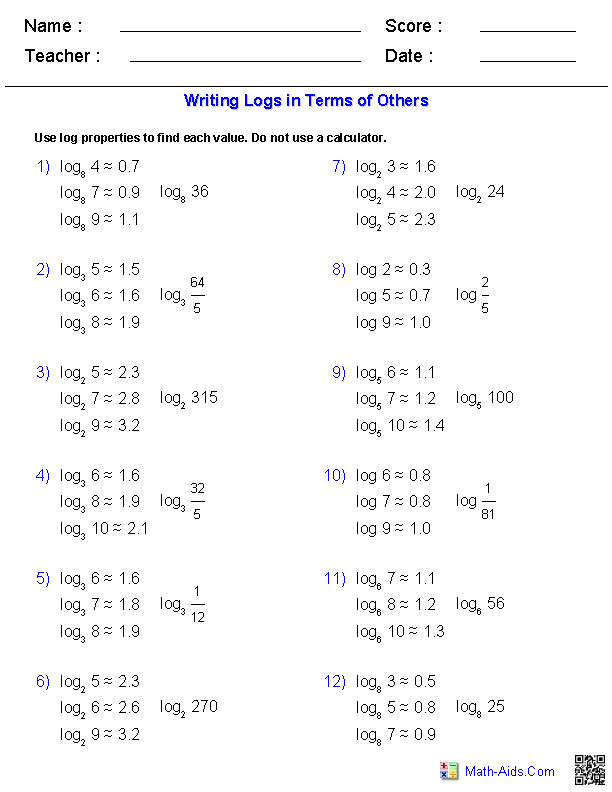##### Logarithmic EquationsWorksheets##### Inverse Functions andLogarithms Worksheets##### Exponential EquationsNot Requiring LogarithmsWorksheets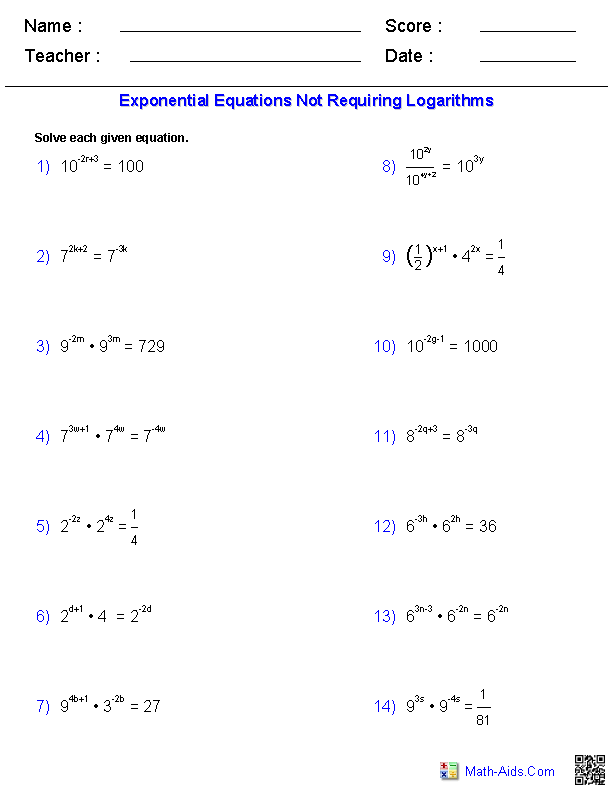##### Exponential EquationsRequiring LogarithmsWorksheets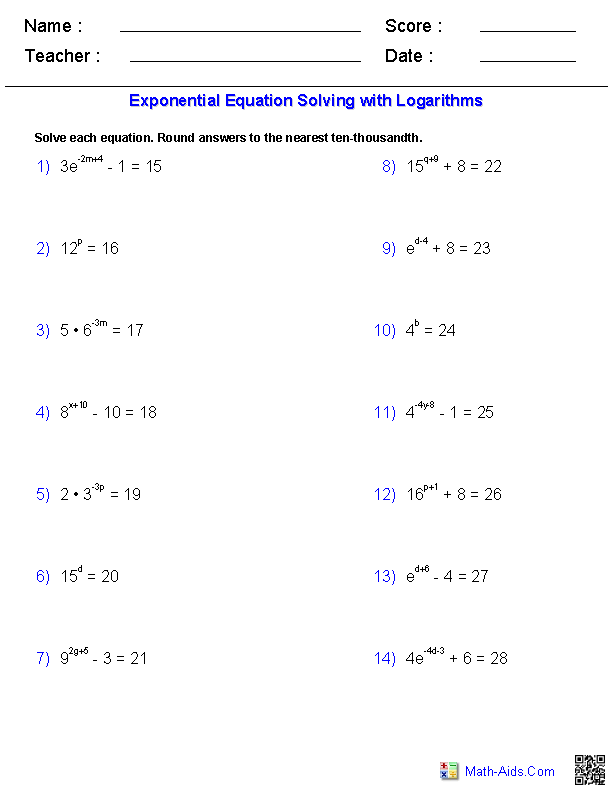##### Graphing LogarithmsWorksheets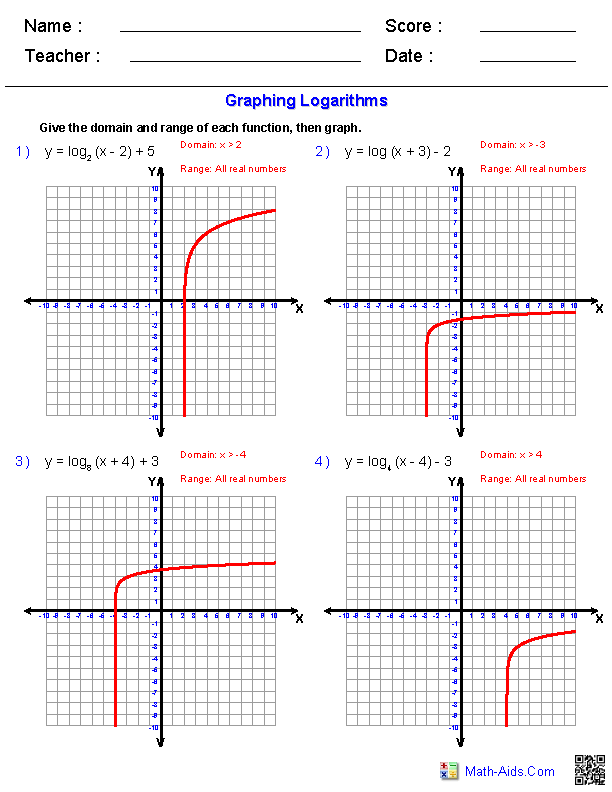##### Graphing ExponentialFunctions Worksheets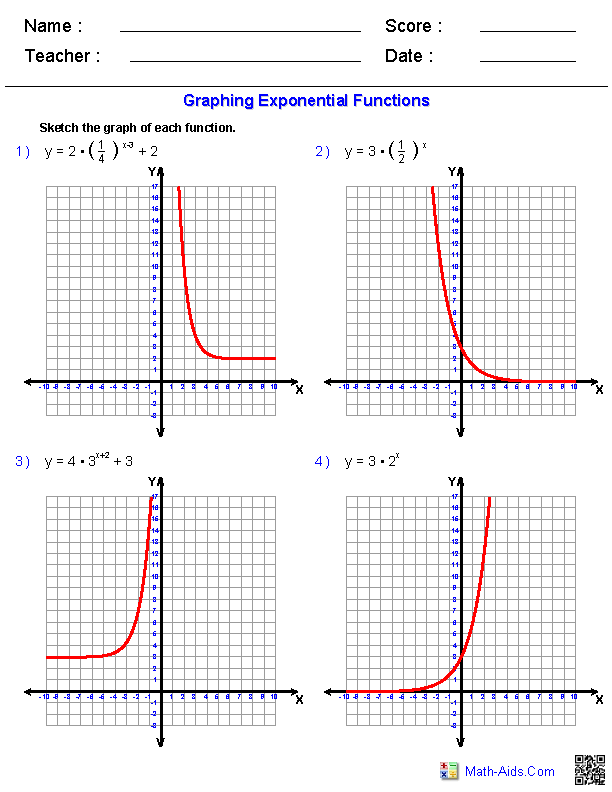Recommended Videos

## Detailed Description for All Exponential and Logarithmic Functions Worksheets

Exponents Properties Handout
These Algebra 2 - Exponential and Logarithmic Functions Worksheets will produce a handout to define and give examples for the different properties of exponents. These Exponential and Logarithmic Functions Worksheets are a good resource for students in the 8th Grade through the 12th Grade.

The Meaning of Logarithms Worksheets
These Algebra 2 - Exponential and Logarithmic Functions Worksheets will give you equations to rewrite into either exponential or logarithmic form. You can chose the form of the problems. These Exponential and Logarithmic Functions Worksheets are a good resource for students in the 8th Grade through the 12th Grade.

Properties of Logarithms Worksheets
These Algebra 2 - Exponential and Logarithmic Functions Worksheets will give you logarithmic expressions to condense and expand. You can chose the form of the problems. These Equations Worksheets are a good resource for students in the 8th Grade through the 12th Grade.

Change of Base Formula Worksheets
These Algebra 2 Exponential and Logarithmic Functions Worksheets will give you logarithmic functions to solve. You can chose the number of problems you want and to what place to round the answers. These Exponential and Logarithmic Functions Worksheets are a good resource for students in the 8th Grade through the 12th Grade.

Writing Logs in Terms of Others Worksheets
These Algebra 2 Exponential and Logarithmic Functions Worksheets will give you exponential equations to solve. You can chose the number of problems you want and the form of the problems. These Exponential and Logarithmic Functions Worksheets are a good resource for students in the 8th Grade through the 12th Grade.

Logarithmic Equations Worksheets
These Algebra 2 Exponential and Logarithmic Functions Worksheets will give you exponential equations to solve. You can chose the form of the problems. These Exponential and Logarithmic Functions Worksheets are a good resource for students in the 8th Grade through the 12th Grade.

Inverses of Logarithms Worksheets
These Algebra 2 Exponential and Logarithmic Functions Worksheets will give you exponential equations to solve. You can chose the form of the problems. These Exponential and Logarithmic Functions Worksheets are a good resource for students in the 8th Grade through the 12th Grade.

Exponential Equations Not Requiring Logarithms Worksheets
These Algebra 2 Exponential and Logarithmic Functions Worksheets will give you exponential equations to solve. You can chose the number of problems you want and the form of the problems. These Exponential and Logarithmic Functions Worksheets are a good resource for students in the 8th Grade through the 12th Grade.

Exponential Equations Requiring Logarithms Worksheets
These Algebra 2 Exponential and Logarithmic Functions Worksheets will give you exponential equations to solve. You can chose the number of problems you want and the form of the problems. These Exponential and Logarithmic Functions Worksheets are a good resource for students in the 8th Grade through the 12th Grade.

Graphing Logarithms Worksheets
These Algebra 2 Exponential and Logarithmic Functions Worksheets will give you logarithmic functions to graph. These Exponential and Logarithmic Functions Worksheets are a good resource for students in the 8th Grade through the 12th Grade.

Graphing Exponential Functions Worksheets
These Algebra 2 Exponential and Logarithmic Functions Worksheets will give you logarithmic functions to graph. You may choose to graph an equation or write an equation from a graph. These Exponential and Logarithmic Functions Worksheets are a good resource for students in the 8th Grade through the 12th Grade.